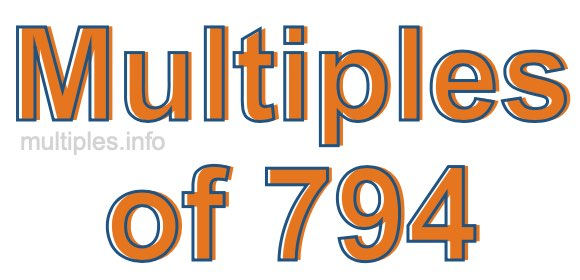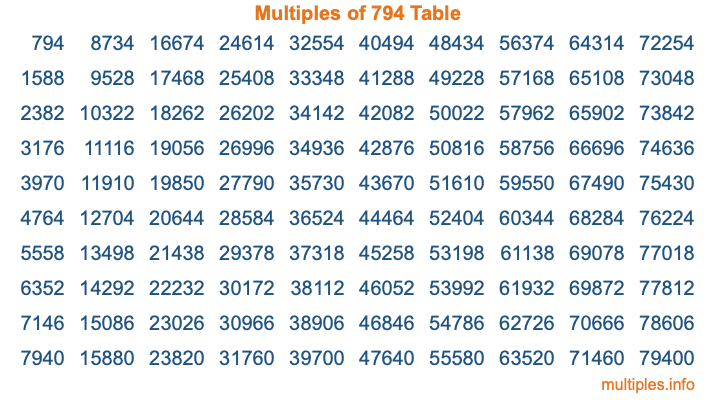Multiples of 794Welcome to the Multiples of 794 page. Here we will first teach you everything you will ever need to know about the multiples of 794, and then give you a study guide summary of everything we taught you to make sure you remember it all. Use this page to look up facts and learn information about the multiples of 794. This page will make you a multiples of seven hundred ninety-four expert!

Definition of Multiples of 794
Multiples of 794 are all the numbers that when divided by 794 equal an integer. Each of the multiples of 794 are called a multiple. A multiple of 794 is created by multiplying 794 by an integer.

Therefore, to create a list of multiples of 794, you start with 1 multiplied by 794, then 2 multiplied by 794, then 3 multiplied by 794, and so on for as long as you want. Thus, the list of the first five multiples of 794 is 794, 1588, 2382, 3176, and 3970. To see a larger list of multiples of 794, see the printable image of Multiples of 794 further down on this page. We also have a category where you can choose any nth multiple of 794.

Multiples of 794 Checker
The Multiples of 794 Checker below checks to see if any number of your choice is a multiple of 794. In other words, it checks to see if there is any number (integer) that when multiplied by 794 will equal your number. To do that, we divide your number by 794. If the the quotient is an integer, then your number is a multiple of 794.

Is  a multiple of 794?

Least Common Multiple of 794 and ...
A Least Common Multiple (LCM) is the lowest multiple that two or more numbers have in common. This is also called the smallest common multiple or lowest common multiple and is useful to know when you are adding our subtracting fractions. Enter one or more numbers below (794 is already entered) to find the LCM.

Check out our LCM Calculator if you need more details about the Least Common Multiple or if you need the LCM for different numbers for adding and subtraction fractions.

nth Multiple of 794
As we stated above, 794 is the first multiple of 794, 1588 is the second multiple of 794, 2382 is the third multiple of 794, and so on. Enter a number below to find the nth multiple of 794.

th multiple of 794

Multiples of 794 vs Factors of 794
794 is a multiple of 794 and a factor of 794, but that is where the similarities end. All postive multiples of 794 are 794 or greater than 794. All positive factors of 794 are 794 or less than 794.

Below is the beginning list of multiples of 794 and the factors of 794 so you can compare:

Multiples of 794: 794, 1588, 2382, 3176, 3970, etc.

Factors of 794: 1, 2, 397, 794

As you can see, the multiples of 794 are all the numbers that you can divide by 794 to get a whole number. The factors of 794, on the other hand, are all the whole numbers that you can multiply by another whole number to get 794.

It's also interesting to note that if a number (x) is a factor of 794, then 794 will also be a multiple of that number (x).

Multiples of 794 vs Divisors of 794
The divisors of 794 are all the integers that 794 can be divided by evenly. Below is a list of the divisors of 794.

Divisors of 794: 1, 2, 397, 794

The interesting thing to note here is that if you take any multiple of 794 and divide it by a divisor of 794, you will see that the quotient is an integer.

Multiples of 794 Table
Below is an image of the first 100 multiples of 794 in a table. The table is in chronological order, column by column. The first column has the first ten multiples of 794, the second column has the next ten multiples of 794, and so on.The Multiples of 794 Table is also referred to as the 794 Times Table or Times Table of 794. You are welcome to print out our table for your studies.

Negative Multiples of 794
Although not often discussed or needed in math, it is worth mentioning that you can make a list of negative multiples of 794 by multiplying 794 by -1, then by -2, then by -3, and so on, to get the following list of negative multiples of 794:

-794, -1588, -2382, -3176, -3970, etc.

Multiples of 794 Summary
Below is a summary of important Multiples of 794 facts that we have discussed on this page. To retain the knowledge on this page, we recommend that you read through the summary and explain to yourself or a study partner why they hold true.

There are an infinite number of multiples of 794.

A multiple of 794 divided by 794 will equal a whole number.

794 divided by a factor of 794 equals a divisor of 794.

The nth multiple of 794 is n times 794.

The largest factor of 794 is equal to the first positive multiple of 794.

794 is a multiple of every factor of 794.

794 is a multiple of 794.

A multiple of 794 divided by a divisor of 794 equals an integer.

794 divided by a divisor of 794 equals a factor of 794.

Any integer times 794 will equal a multiple of 794.

Multiples of a Number
Here you can get the multiples of another number, all with the same attention to detail as we did for multiples of 794 on this page.

Multiples of
Multiples of 795
Did you find our page about multiples of seven hundred ninety-four educational? Do you want more knowledge? Check out the multiples of the next number on our list!